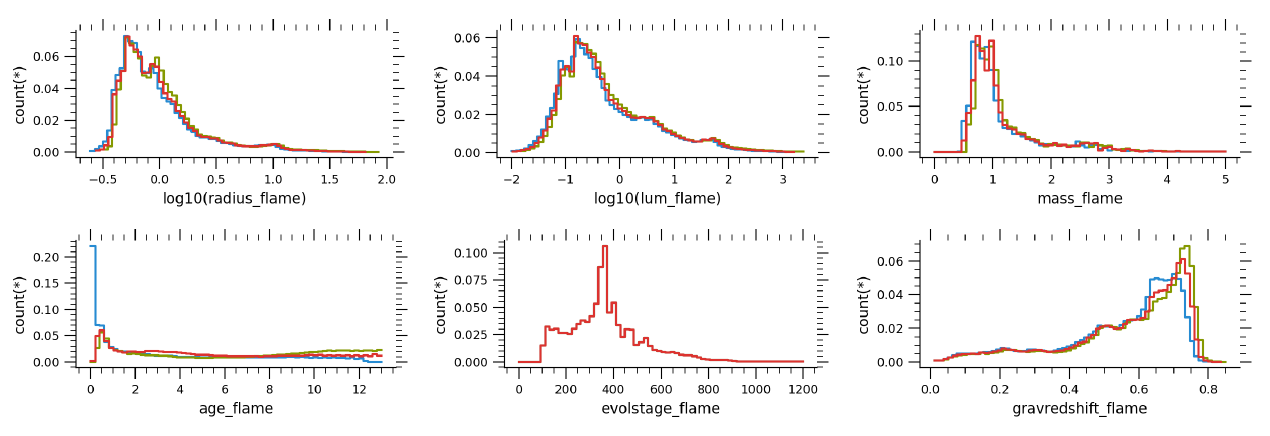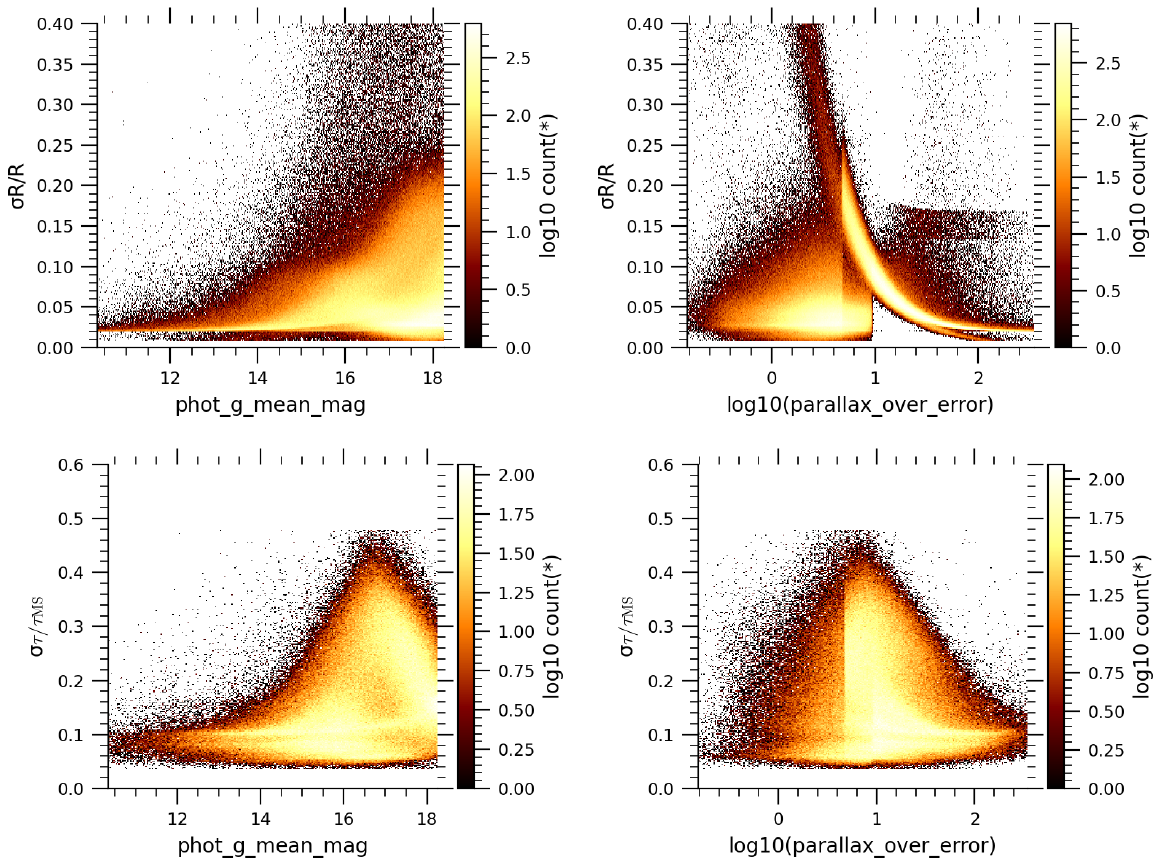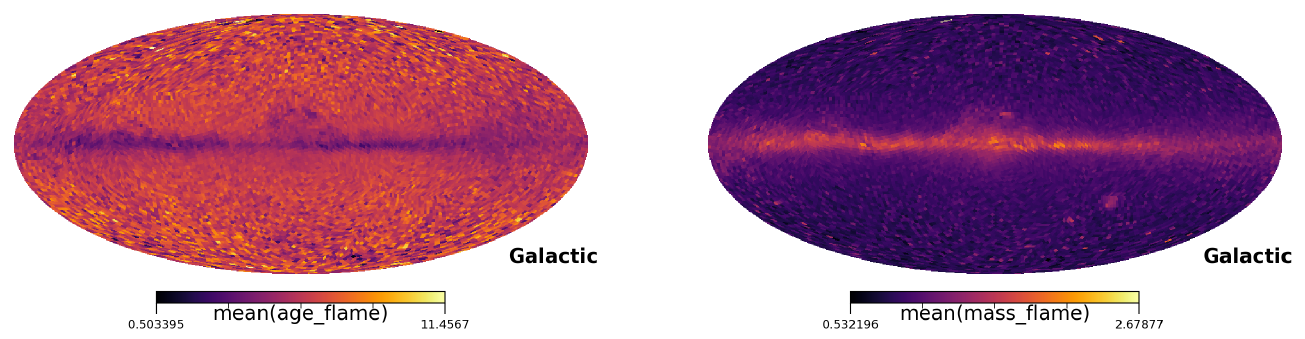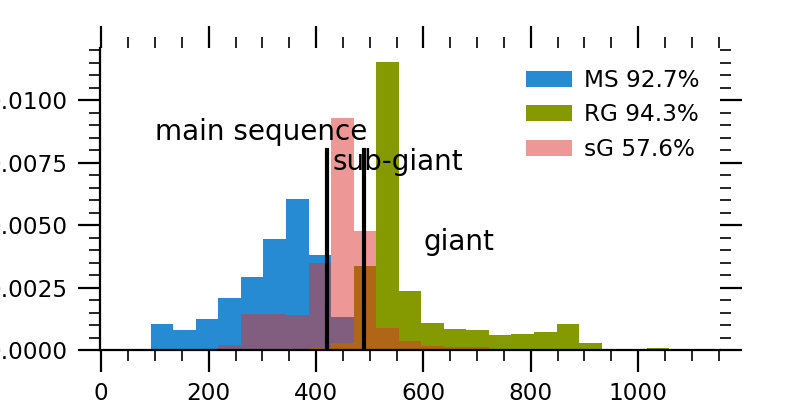# 11.3.6 Final Luminosity Age Mass Estimator (FLAME)

Author(s): Orlagh L. Creevey, Frédéric Thévenin

## Goals

The Final Luminosity Age Mass Estimator, FLAME, aims to produce the stellar mass and evolutionary parameters for each Gaia source. These parameters comprise the radius ${\cal R}$, luminosity ${\cal L}$, gravitational redshift $rv_{\rm GR}$, mass ${\cal M}$, age $\tau$, evolutionary stage $\epsilon$, and auxiliary parameters. They use the output from GSP-Phot and GSP-Spec, along with astrometry, photometry and stellar models.

FLAME results in Gaia DR3 based on GSP-Phot parameters are found in the astrophysical_parameters table for approximately 300M sources ($G<18.25)$. A second result is produced only for the bright sources based on GSP-Spec parameters for approximately 5M sources, and these are found in the astrophysical_parameters_supp table. We note that these latter FLAME results are based on the GSP-Spec results that appear in the astrophysical_parameters table, and not those in the astrophysical_parameters_supp table.

## Input data and models

FLAME requires atmospheric parameters ($T_{\rm eff}$, $\log g$, $[\rm M/H]$) to derive a bolometric correction $\mathrm{BC}_{G}$, see Section 11.2.3. Then, using extinction $A_{\rm G}$, magnitude and an estimate of the distance, ${\cal L}$ is derived from the standard equation

 $M_{G}=-2.5\log\mathcal{L}+M_{\rm bol,\odot}-BC_{G}.$ (11.26)

Here $M_{G}$ is calculated using phot_g_mean_mag, ag_gspphot and either (1) the parallax or (2) the distance_gspphot, (see flags_flame for details). ${M}_{\rm bol,\odot}=4.74$ mag, see Section 1.2.3, and $\mathrm{BC}_{G}$ is based on the MARCS atmosphere models, see Creevey et al. (2023) for details and Section 11.2.3.

${\cal R}$ is then derived from the Stefan-Boltzmann equation

 $\mathcal{L}=\mathcal{R}^{2}(T_{\rm eff}/T_{\rm eff,\odot})^{4}$ (11.27)

where $T_{\rm eff,\odot}=5772$ K (see Section 1.2.3), and ${\cal R}$ and ${\cal L}$ are given in solar units. The parameter $rv_{\rm GR}$ is then obtained using ${\cal R}$ and the input $\log g$. Finally, ${\cal M}$, $\tau$ (age), and $\epsilon$ (only two of these are independent) are then obtained by comparing ${\cal L}$ and $T_{\rm eff}$ with the BASTI (Hidalgo et al. 2018) solar metallicity stellar evolution models. The parameter $\epsilon$ is an integer from 100 to typically 1200 representing the evolution time step of the stellar model of a particular mass, see evolstage_flame for details.

## Method

FLAME employs a Monte Carlo bootstrap method to derive its parameters and uncertainties. For the GSP-Phot-based parameters, each element of the Markov Chain Monte Carlo (MCMC) is processed by FLAME, so correlations are preserved. For the GSP-Spec-based ones, the input parameters are perturbed $N=300$ times based on the reported asymmetric uncertainties. For each $i$ iteration, ${\cal L}$, ${\cal R}$, and $rv_{\rm GR}$ are derived as described in the paragraph above. The comparison with the stellar models to derive ${\cal M}$, $\tau$, $\epsilon$ is based on a linear least-squares method which optimises the likelihood function for ${\cal L}$ and $T_{\rm eff}$.

For each source, distributions of parameters are produced and weighted statistics are calculated, where the weights depend on the position of the star in the HR diagram. The weighted median (50th percentile) value is reported as the stellar parameter, and the 16 and 84 percentile values are reported as the lower and upper confidence intervals, respectively.

## Output

The FLAME products in Gaia DR3 comprise ${\cal L}$, ${\cal R}$, and $rv_{\rm GR}$, ${\cal M}$, and age, which are accompanied by a lower and upper confidence interval. ${\cal L}$, ${\cal R}$, and ${\cal M}$ are given in solar units, age in Gyear, and $rv_{\rm GR}$ in $\rm\,km\,s^{-1}$. The evolution stage is also derived and this is an integer typically between 101 and 1200 indicating which stage of the evolution the star is in: 100 - 420 for a main sequence star and $>490$ for a giant, see the detailed description in evolstage_flame. Two auxiliary parameters are also published in Gaia DR3: (1) $\mathrm{BC}_{G}$ and (2) flags, c.f. Table 11.26, see next paragraph.

The flag (of type string) flags_flame* contains either two (GSP-Phot-based) or one (GSP-Spec-based) characters which denote the following: (A) The first character informs the user about the quality of the mass and age: (0) nothing to report, (1) the star is a giant and the mass, age and uncertainties need to be used with care, (2) no mass/age is provided for this star. (B) The second character informs the user if the parallax (0, 2) or the distance (1) has been used to estimate ${\cal L}$. In general the parallax is used in the following cases (flag = 0): if (1/parallax $<2000$ and parallax_over_error $>5$) or (1/parallax $>2000$ and parallax_over_error $>$ 10), while distance_gspphot is used otherwise (flag = 1). These values have been chosen based on validation of the results. However, some convergence issues have been reported with distance, and the parallax is then used by default (flag = 2), see Section 11.3.3 (5) for details of the convergence issues which are not related to the atmospheric properties. As an example, flags_flame = ’10’ implies that (A) the star is most likely a giant, so treat the mass and age with care, and (B), the luminosity (and all other parameters) used the parallax and not distance_gspphot.

flags_flame_spec informs the user uniquely about the mass and age, and therefore takes values of either ’0’, ’1’, or ’2’. Table 11.26 summarises the number statistics for each of these flags.

## Scope

FLAME processed all sources that produced a result by GSP-Phot (BP/RP-based) for sources with $G$ $<18.25$. The limit in magnitude was imposed due to the tight processing and validation schedule. It also processed all sources that produced a result by GSP-Spec (RVS-based) for $G$ $<15$. After post processing (see below), which removed invalid results and set flags for the data archive, there are total of 284 666 978 flame results in the astrophysical_parameters table, and 5 075 602 results in the astrophysical_parameters_supp table. However, not all parameters are available for all sources; typically, mass and age are available for approximately half of this number, see Table 11.27 for details.

During postprocessing the following filters were imposed to remove FLAME results that were shown not to be reliable from the astrophysicalParameters table:

• if parallax_over_error $<$ 0.5

• teff_gspphot outside of the valid range: 2 500 – 20 000 K

• azero_gspphot outside of the valid range: 0 – 10 mag.

• mh_gspphot $<-3.5$ dex

• mass_flame, age_flame, evolstage_flame removed if models failed to converge for more than 80% of the iterations. Other parameters were kept.

• age_flame removed if outside of the valid range: 0.1 - 13.5 Gyr

During post processing the following filters were imposed for FLAME parameters which removed unreliable results for sources from the astrophysical_parameters_supp table:

• if parallax_over_error $<$ 0.5

• teff_gspspec outside of the valid range: 4500 – 7500 K

• azero_gspphot outside of the valid range: 0 – 10 mag.

• mass_flame_spec, age_flame_spec, evolstage_flame_spec removed if models failed to converge for more than 80% of the iterations. Other parameters were kept.

• age_flame_spec removed if outside of the valid range: 0.1 - 13.5 Gyr

## Results

### Distributions of parameters.

In Figure 11.28 we show the distribution of the main stellar parameters from FLAME for a random subset of 2 000 000 stars in Gaia DR3. The sources with FLAME parameters comprise approximately 88% main sequence stars and only about 6% giants (the rest are subgiants). The lower confidence interval (blue) of age_flame peaks near zero and this indicates large uncertainties in this parameter.

Figure 11.29 shows the distributions of uncertainties, as a function of magnitude and relative parallax precision for the same random subset. The uncertainties in the parameters generally increase with both of these. The uncertainties for ${\cal L}$ follow the same trend as that of the ${\cal R}$. On the right panels, we can see primarily two sets of results, one set shows uncertainties which increase with decreasing parallax_over_error. These are the stars whose second flag = ’0’, i.e. which used the parallax information to estimate the distance. The second group on that same panel, but towards lower parallax_over_error has a more or less constant relative radius precision, and these are the stars that used distance_gspphot as the distance estimate. The lower panels of Figure 11.29 show the relative precision of age as a function of the estimated main sequence lifetime. These uncertainties vary much smoother with magnitude and relative parallax precision, because they are constrained by the stellar models. A similar result is found for the mass.Figure 11.28: Distributions of FLAME parameters in the astrophysical_parameters table. Red, blue, and green indicate respectively, the value of the parameter, the lower and upper confidence interval.Figure 11.29: Distributions of FLAME uncertainties as a function of G and parallax relative precision. The top panels show the results for the radius and a similar trend is seen for the luminosity (not shown). The lower panels show the relative precision of age compared to its main sequence age, for main sequence stars only. The relative precision in mass follows a similar trend to the age.

### Astrophysical interpretation.

To verify the integrity of the FLAME parameters, we show the HR diagram using lum_flame and teff_gspphot using the full 2 000 000 sources in Figure 11.30 left panel (all flags), and then selecting on flags_flame = ’00’ or ’10’ or ’20’ i.e. parameters using only the parallax. The position of the stars in this diagram make astrophysical sense. By comparing the left and right panels, we can see that by removing the stars with worse parallax errors (which used distance_gspphot) the diagram removes certain artefacts. The artefacts that one can see on the left panel, does not imply that these are incorrect, or errors, simply that these sources have lower quality for FLAME, and should be used best in a statistical sense or to find interesting targets, rather than on a source-by-source basis. In this figure, the colour-code indicates density of sources, where the main sequence is clearly populated by the expected $\sim$90% of stars.

Figure 11.31 shows similar HR diagrams but colour-coded by left: mass_flame, centre: age_flame and right: evolstage_flame. In the three figures the trends are as we expect, with mass increasing in general from bottom to top, with the most massive stars much more evolved (orange colour at tip of the giant branch), the mean age increasing diagonally up the HR diagram, and the evolution stage increasing from low luminosity to high luminosities. On the right-most panel, there is a set of sources colour-coded by evolstage_flame with values larger than 1300; these sources have been shown to have low SNR XP spectra and the APs from GSP-Phot are probably incorrect . This result was found during the internal validation process. Figures using GSP-Spec-based parameters show similar results.Figure 11.30: The HR diagram using lum_flame and teff_gspphot. Left: No selection on flags_flame. Right: Selecting on flags_flame = ’00’ or ’10’ or ’20’.Figure 11.31: The HR diagram using lum_flame and teff_gspphot, colour-coded by mass_flame (left), age_flame (centre), and evolstage_flame (right) and selecting on flags_flame = ’00’ or ’10’ or ’20’.Figure 11.32: Galactic projection of mass_flame and age_flame at HEALPix level 5.

In Figure 11.32 a galactic projection of the mean mass and mean age at HEALPix level 5 in shown. It can be seen that lower masses and older ages are found away from the Milky Way disk, while higher masses are found primarily in the galactic disk.

### Comparison with external catalogues.

In Figure 11.33 – Figure 11.35, we explain and show comparisons of FLAME parameters with those from external catalogues, while in Table 11.29 we give a quantitative comparison for the radius, mass and age. Extensive comparisons was done with all parameters during internal validation of FLAME and one should refer to Section 11.4.5 and Fouesneau et al. (2023) for further validation.

In general we find good agreement for all FLAME parameters with some systematic differences on the order of a few % at most with external catalogues. The parameters that we recommend that users heed with caution are the mass and age for evolved stars, where generally the uncertainties are much larger, but also the comparison with external catalogues is not always satisfactory. Concerning these sources, we have decided to flag these with ”1” in the first character of flags_flame. Best results with external catalogues for mass and age of evolved stars with flags_flame = ’10’ or ’11’ or ’12’ is found in the mass range of $\sim$1 – 2 $M_{\odot}$.Figure 11.33: Comparison of radius_flame with asteroseismic analyses from (left) Serenelli et al. (2017) for 306 main sequence stars and (right) Pinsonneault et al. (2018) for 3 194 giants. Median differences are at the 3% and –1% level, respectively.Figure 11.34: Left: Comparison of mass_flame with Casagrande et al. (2011) for 5 926 stars. Median differences are at the <1% level. Right: Comparison of age_flame with Serenelli et al. (2017) and Creevey et al. (2017).Figure 11.35: Distribution of evolstage_flame values with sources in common with Berger et al. (2018) for 151 670 stars. The colour-code indicates the evolution stage from the external catalogue, and the x-axis shows the value of evolstage_flame. The numbers in the legend indicate the purity, i.e. when evolstage_flame is in agreement with the values given by Berger et al. (2018).

### Comparison of mass and age with an external code.

To further validate the results, we compared FLAME output with that from an independent public code, SPiNs (Lebreton and Reese 2020), focusing specifically on the derivation of masses, ${\cal M}$, and ages $\tau$. We limited our comparison to random sets of 600 stars that cover different ranges of magnitudes while only requiring that the parallax relative uncertainty is better than 50%. The input $T_{\rm eff}$, ${\cal L}$, and $[\rm M/H]$, were provided to both codes and the comparison of results for two representative samples is shown in Figure 11.36. As can be seen, FLAME and SPiNS have very similar performance, and agreement to within 1-$\sigma$ is found for main sequence mass and age.Figure 11.36: Comparison of mass_flame for stars with G∼15 (left) and age_flame for stars with G∼9 (right) with a publicly available code, SPiNS. The colour code is indicative of evolution stage. We find good agreement for main sequence stars (high log⁡g and low evolstage_flame). Differences are seen primarily for the more evolved giants. No error bars are shown in these figures, but agreement is at the 3-σ level.

## Use

FLAME results can be employed for a wide range of objectives starting from sample selection to direct analysis of individual stars. However, the following caveats should be noted, in particular for analyses on individual stars:

(1) mass, age and evolution stage are derived by comparing ${\cal L}$ and $T_{\rm eff}$ to solar metallicity stellar models.
(2) the uncertainties in the mass and age are based on comparison with solar metallicity models, no contribution to the uncertainty is given for a non-solar metallicity star.
(3) Postprocessing information which filtered results may need to be considered depending on the use case, see Section 11.3.6.
(4) Best results are obtained by considering the flags ’00’, ’10’, or ’20’, i.e. second digit = ’0’.
(5) For the masses and ages of giants (first character in flag=’1’), results should only be used in the 1 – 2 $\,{\cal M}_{\odot}$ range when age $>$ 1 Gyr (roughly 14M objects).
(6) The uncertainties have been shown to be underestimated. These uncertainties are based only on the input data and do not consider sources of external errors, i.e. a radius or luminosity with $<1\%$ uncertainty most likely has a very high signal-to-ratio spectra and parallaxes.
(7) As the FLAME models only consider masses between 0.5 – 10 $\,{\cal M}_{\odot}$, and evolution stages from the Zero-age-main-sequence to the tip of the giant branch, any source with observed parameters outside of this parameter space will receive incorrect mass, age, and evolution stage values. Many of these sources have already been removed due to lack of convergence, but some values still remain.

The following inconsistencies are known:
(1) FLAME processed the mean value of GSP-Spec-output, however, this mean value was updated to the median during the GSP-Spec post processing. This could affect a few outliers, but overall results should not be affected.
(2) There are 153 474 lum_flame without bc_flame. The FLAME parameters are valid. The missing $\mathrm{BC}_{G}$ has been traced back to a minor code issue that has been reproduced, checked, and fixed. This has been reported in the known issues page. These numbers are recoverable by using the bolometric correction function (Creevey et al. 2023) which is available on the Gaia DR3 software and tools webpages.